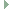OGRE  13.6 Object-Oriented Graphics Rendering Engine
Ogre::ScaleControllerFunction Class Reference

Predefined controller function which simply scales an input to an output value. More...

#include <OgrePredefinedControllers.h>Inheritance diagram for Ogre::ScaleControllerFunction:

## Public Member Functions

ScaleControllerFunction (Real scalefactor, bool deltaInput)

Real calculate (Real source) overridePublic Member Functions inherited from Ogre::ControllerFunction< Real >
ControllerFunction (bool deltaInput)
Constructor. More...

virtual ~ControllerFunction ()

## Static Public Member Functions

static ControllerFunctionRealPtr create (Real scalefactor, bool deltaInput=false)
Constructor, requires a scale factor. More...

## Detailed Description

Predefined controller function which simply scales an input to an output value.

## ◆ ScaleControllerFunction()

 Ogre::ScaleControllerFunction::ScaleControllerFunction ( Real scalefactor, bool deltaInput )

## ◆ create()

 static ControllerFunctionRealPtr Ogre::ScaleControllerFunction::create ( Real scalefactor, bool deltaInput = false )
inlinestatic

Constructor, requires a scale factor.

Parameters
 scalefactor The multiplier applied to the input to produce the output. deltaInput If true, signifies that the input will be a delta value such that the function should add it to an internal counter before calculating the output.

## ◆ calculate()

 Real Ogre::ScaleControllerFunction::calculate ( Real source )
overridevirtual

The documentation for this class was generated from the following file: